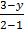### Sample Problem

The slope of the line is 3, and you know it goes through the point (2, 3). Another point on the line goes through the x-coordinate 1. Find the missing y-coordinate:

(1, )

#### Solution

We can plug in the numbers we know into the formula for the slope of a line and solve for the unknown y-coordinate.

3 =3 =3 = 3 – y

y = 0